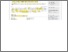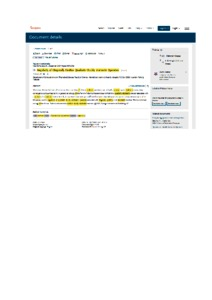# On regularity of diagonally positive quadratic doubly stochastic operators

Saburov, Mansoor (2017) On regularity of diagonally positive quadratic doubly stochastic operators. Results in Mathematics, 72 (4). pp. 1907-1918. ISSN 1422-6383PDF - Published Version
Restricted to Repository staff onlyPreview
PDF (SCOPUS)

## Abstract

The classical Perron–Frobenius theorem says that a trajectory of a linear stochastic operator associated with a positive square stochastic matrix always converges to a unique fixed point. In general, an analogy of the Perron–Frobenius theorem does not hold for a quadratic stochastic operator associated with a positive cubic stochastic matrix. Namely, its trajectories may converge to different fixed points depending on initial points or may not converge at all. In this paper, we show regularity of quadratic doubly stochastic operators associated with diagonally positive cubic stochastic matrices. This is a nonlinear analogy of the Perron–Frobenius theorem for positive doubly stochastic matrices.

Item Type: Article (Journal) 6769/59920 Quadratic doubly stochastic operator, cubic stochastic matrix, regularity. Q Science > QA Mathematics Kulliyyah of Science > Department of Computational and Theoretical Sciences Dr Mansoor Saburov 04 Dec 2017 11:42 13 Mar 2018 16:24 http://irep.iium.edu.my/id/eprint/59920View Item